short communicationsJOURNAL OFSYNCHROTRONRADIATION
ISSN: 1600-5775
Volume 20| Part 1| January 2013| Pages 190-193

Concepts for flexible and efficient monochromatization of X-rays by refraction to a relative bandwidth of the order of 0.5%

aSincrotrone Trieste ScpA, SS 14 km 163.5, I-34149 Basovizza (TS), Italy
*Correspondence e-mail: werner.jark@elettra.trieste.it

(Received 14 June 2012; accepted 4 October 2012; online 10 November 2012)

Recently it was shown experimentally that regular arrays of tiny prisms can be used as X-ray monochromators providing a spectral bandwidth of below 2%. Successively the measured spectral transmission functions of monochromators operated under different conditions were found to be in agreement with expectations within an analytical model. This type of monochromator focuses chromatically and thus necessitates the use of an exit slit for the monochromatization. This contribution will show that the lower limit for the achievable bandpass can be predicted under practically feasible experimental conditions. Refractive monochromators based on prism arrays are found to be feasible solutions for monochromatization with high transmission to a spectral bandwidth of the order of 0.5%. The bandwidth can easily be increased by adjusting the exit slit setting accordingly. Consequently, the presented refractive devices would make for tunable monochromators with tunable bandwidth, which provides more flexibility for an intermediate bandwidth of <1%, which multilayer monochromators have difficulty providing routinely.

1. Introduction

When monochromatic X-rays are required in an experiment, the monochromatization is almost always made using diffraction. Diffraction crystals (see, for example, Matsushita, 1983) provide the smallest relative bandwidths with values of the order of ΔE/E ≃ 2 × 10−4 or smaller. More flux is usually obtained when multilayer coatings (see, for example, Underwood & Barbee, 1981), i.e. artificially produced two-dimensional layered structures with larger periodicity, are used with high transmission and with significantly larger bandwidths of the order of a few percent. The intermediate range of bandwidths of the order of ΔE/E ≃ 3 × 10−3 requires multilayers with several hundred bi-layers and smaller periodicity, as proposed and tested by Morawe et al. (2001) and Englich et al. (2005). The latter showed that the reported increase in bandwidth compared with diffraction crystals is compatible with the needs of high-resolution crystallography experiments, and could thus make full use of the related increase in photon flux. As the related multilayer mirrors are very demanding objects, the search for alternatives is on-going. Consequently, the suitability of refraction, which has already been used for centuries for the dispersion of visible light in prisms (see, for example, Newton, 1704), has been discussed more recently for the monochromatization.

Refraction will disperse the photon energies in angle, and thus for their discrimination an exit slit needs to be employed. For ease of use in a monochromator it would be desirable that the slit remains stationary while tuning the photon energy. Such flexibility cannot be achieved with the first generation of refractive and chromatic X-ray lenses in the form of stacks of concave lenses as first described by Snigirev et al. (1996). Consequently, the refractive monochromator option started to be discussed. Cederström et al. (2000, 2001) had found in the (inclined) sawtooth refractive lens a concept for a continuously zoomable X-ray lens. They, and later Jark (2004), predicted feasible bandwidths of the order of 3%, i.e. a performance similar to multilayer mirrors. After that, Fredenberg et al. (2009) described the first monochromatization experiments using prism arrays with a laboratory X-ray source. More recently, Liu et al. (2012) systematically measured the performance of this class of X-ray focusing devices as X-ray monochromators for synchrotron radiation; a promising bandwidth below 2% was achieved. The best performance was reported when an absorbing wire obstructed the central part of the lens, where the deflection angle is small. Successively, Jark (2012) showed that this result is in agreement with rigorous calculations. These calculations, as well as the experimental data, showed that the transmission functions for centrally obstructed prism array lenses are almost trapezium-shaped, i.e. the sloped part of the function is relatively small compared with the width of the function. Tails are almost completely absent. This and the freedom from higher-order radiation are very advantageous features of an X-ray monochromator. Jark (2012) discussed that the prism array lens can easily provide photon energy tuning in a fixed exit slit when it is rotated in the yaw angle α, i.e. around an axis in the dispersion plane and orthogonal to the incident beam as shown at the top of Fig. 1. This monochromator, with only a single component, will simultaneously monochromatize and focus on-axis in a stationary image. For a reasonable rotation range of Δα = 65°, the photon energy can be varied by at least ∼50%, e.g. between 17 and 25.5 keV. The purpose of this study is to identify in which bandwidth range such a monochromator can provide a competitive performance. In any case the refraction makes any related monochromator concept rather flexible, as the bandwidth is always easily variable by use of a variable-slit setting. Then one can advantageously always trade bandwidth for an increase in photon flux.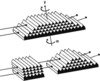Figure 1 Top: proposal for an X-ray monochromator based on a prism array half lens, in which tuning is provided by rotating by angle α around an axis y orthogonal to the incident beam as shown. Only the illuminated prisms in an off-axis prism lens are drawn. Bottom: decomposition of the prisms in the illuminated area into two structures, which perform optically identically.

2. Discussion of the expected optimum' bandwidth performance

The prism array lens was originally proposed by Jark et al. (2004b) as a kinoform for X-rays, i.e. as a structure which manipulates the transmitted phase of the light for focusing and without the introduction of phase discontinuities. The primary purpose of this solution was the reduction of the absorption in the structure, as the apertures of other refractive X-ray lenses with concave surfaces are absorption limited. With larger apertures the kinoforms can thus provide relatively smaller diffraction-limited focus sizes; however, only for discrete photon energies. Then, according to Jark et al. (2006), in single-photon-energy operation the kinoform could achieve very small bandwidths, comparable with crystal monochromators. This condition goes beyond the scope of this contribution, which will instead discuss more flexible X-ray monochromators. Here the prism arrays will be considered as assemblies of independently focusing microlens systems. These microlenses are then operated with apertures A that are identical to the height of the prisms h. The diffraction-limited spot size i′ for such lenses with focal length f, when operated at a wavelength λ, is approximately i′ ≃ λ(f/A). Liu et al. (2012) operated their prism array with A = h = 12 µm and with f ≃ 0.5 m for λ ≃ 0.08 nm, which results in i′ ≃ 3.3 µm. This size can be matched readily with an exit slit. For optimum performance the ideally demagnified source size i has to be of the same size. This is smaller than the prism height, and consequently the then unavoidable aberrations need to be removed from the focused beam by curving some of the prism side walls, as discussed by Jark et al. (2004b).

The symmetry of the lens allows us to calculate the monochromator performance for a centrally obstructed lens by considering only one of the active off-axis segments in a half lens as shown in the top of Fig. 1. For this lens the spectral transmission function can be calculated analytically as shown by Jark (2012). Now the scope of the present discussion is finding the smallest achievable bandwidth which cannot be derived directly from the previously presented model. For the present discussion it is advantageous to arrange the prisms in the monochromator structure differently. In the off-axis segment of the prism lens monochromator shown in Fig. 1the central prism rows, containing up to M′ prisms per row, are missing. As the total beam deflection angle in any row of a prism array depends linearly on the number of traversed prisms, the decomposed prism structure presented at the bottom of Fig. 1will refract the incident beam identically to the structure at the top. One can then separate the calculation into the treatment of the refraction in the composite prism, with M′ prisms in any row, and into the refraction in the downstream unobstructed half-lens.

The beam deflection Δφ in the composite prism is given by Liu et al. (2012) as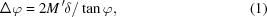where φ is the angle of grazing incidence onto the prism wide walls and δ is the refractive index decrement of the prism material, when the refractive index is written as n = 1 − δ. For X-rays far above the absorption edges of a material one has the dependence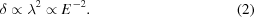Then δ and consequently Δφ vary significantly with photon energy. An observer at the position of the prism lens looking through the composite prism into the direction of the source will then see an extended virtual source at the original source distance p upstream of the prisms, in which the photon energies are dispersed in the vertical direction according to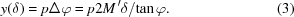The source sizes for different photon energies are identical and the dispersion within the source size s is then given as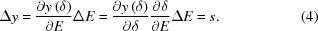By use of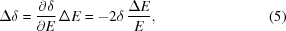one finally obtains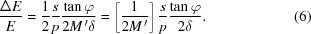In this case s/p is the angular spread of the incident beam owing to the finite source size. The source-size-limited relative spectral bandwidth according to (6)is then simply half of the ratio between the latter angular spread and the beam deflection angle in the composite prism according to (1). In order to make this bandwidth available behind a monochromator, one will have to discriminate a chosen deflection angle. This can be achieved by any focusing device, e.g. by a mirror, in combination with an exit slit. Here this selection will be made by half of a prism lens. It is then interesting to see whether the refraction in the prism lens will further improve the spectral bandwidth provided by the composite prism.

After passage through the composite prism for the downstream prism lens the beams with different photon energies are arriving from vertically dispersed virtual sources. In the prism orientation shown in Fig. 1, according to equations (1)and (2), the dispersion displaces the virtual positions for the source for increasing photon energies downwards. If the lens now focuses all photons emitted from a source of size s with a photon energy E0 into the exit slit of the matched size i, then the virtual sources with differing photon energy but with identical size will be found as shown in Fig. 2. The matching slit size is given by the lens equation, which predicts the size of the ideally demagnified source image at a distance q from the monochromator as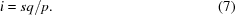For the ease of presentation I will at this point neglect the absorption in the concluding prism lens. Compared with the more rigorous calculations presented by Jark (2012), this assumption underestimates the spectral bandwidth slightly, when the length of the prism lens dominates over the length of the composite prism upstream of it. Instead it is a valid approximation when the size of the composite prism is similar or larger than the prism lens. I will start with a geometrical aperture of the prism lens given by Jmaxh in a lens containing Jmax rows. Then the distance of any ray, which can pass the lens, from the lens optical axis is approximately given by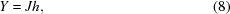for J < Jmax. In a lens with the prism number increment M between adjacent prism rows, the number of prisms in row J is MJ and the deflection angle in this row can be written as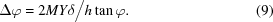Now, rays A and B in Fig. 2indicate for different photon energies, i.e. for E < E0 and for E > E0, respectively, the extreme beam paths with the maximum possible vertical offset in the lens center. All other possible beam paths between the respective sources and the exit slit pass the lens with smaller offset. With the indices A and B for the two extreme rays the related deflection angles are given by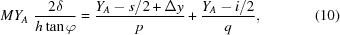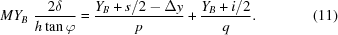Now, we are interested in the two photon energies EA and EB, for which the geometrical aperture is half of the lens height, i.e. we have YA = (Jmax/2)h = YB. Their separation then provides the achievable spectral bandwidth of the monochromator.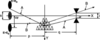Figure 2 Ray paths through a prism lens, when the incident beam is already dispersed in photon energy after passage through a composite prism. The ellipse labelled E0 indicates the position of the virtual source with size s, for which all emitted rays pass the exit slit of opening i to the right. p and q refer to the distances of the lens from the source and from the slit, respectively. The virtual sources of different photon energies (labelled E < E0 and E > E0) are displaced by Δy with respect to the in-focus source. The rays labelled A and B are those rays which cross the optical axis of the lens at the largest offset for a given photon energy.

Using the lens equations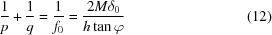and (7)as well as (5)one finally finds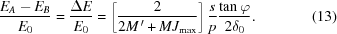This equation contains the same parameters present in equation (6), but has a different prefactor. It now also contains the number of prism rows Jmax in the monochromator and it is identical to the prefactor in (6), when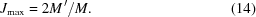Consequently, with this composition the lens part does not improve the spectral bandwidth obtainable by use of the composite prism alone, but exclusively performs a focusing action. Then, in this prism-array monochromator, one-third of the prisms in the last row belong to the composite prism and two-thirds are part of the prism lens. The missing central rows, containing up to M′ prisms, then account for one-third of the geometrical aperture of the related unobstructed lens. Now, both objects, the composite prism and the prism lens, contain the same amount of prisms; thus they roughly absorb the same amount of flux. Then, as far as the efficiency is concerned, it would be better to combine the composite prism with a more efficient mirror for the focusing. On the other hand, the here-discussed solution based on a single device facilitates very much the alignment and the operation. For the further discussion I will consider then that equation (6)presents the ultimately efficiently available minimum spectral bandwidth, or the optimum' bandwidth of a prism-array monochromator. Formally a further improvement is possible by increasing M′ in a combination with a fixed number of prisms in the last row. However, the improvement is rather moderate to, at most, two-thirds of the prediction by use of (6). On the other hand, it will be achieved with an undesirable decrease in the geometrical aperture, as provided by a lens with decreasing number of rows. As the use of a mirror instead of the prism lens permits a completely free choice of the geometrical aperture, this configuration is to be preferred under this condition. In this case all small prisms could be accommodated in a composite prism, which will then be twofold longer, providing a twofold improvement in the bandpass compared with the prediction by use of (6)and with no restriction in the geometrical aperture.

As far as the efficiency of the prism-array monochromator is concerned, one has to recognize that the averaged transmission decreases to an ultimately still acceptable value of 0.44, when the longest optical path in the material in the composite prism is identical to the attenuation length, AL, of the lens material. With the prism base length b this leads to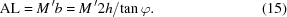The smallest slit is needed when the monochromator is operated with the diffraction-limited spot size for the prism rows. Under this condition the acceptable angular spread in the incident beam can at most be identical to the angular spread of the transmitted beam due to diffraction, i.e. one can put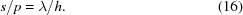Then the ultimate `optimum' bandwidth which a prism-array monochromator can provide with reasonable experimental boundary conditions is given by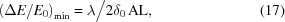which depends only on material properties. The related data for the use of the photoresist SU8 is presented in Fig. 3depending on the photon energy and for the optical constants for SU8 as presented by Jark (2012). We see that bandwidths below 1% and down to about 0.3% are possible in prism-array monochromators. By use of twofold-longer composite prisms in combination with focusing mirrors, twofold-smaller bandwidths are possible for unchanged transmissions, i.e. for an averaged transmission of 44% in the composite prism.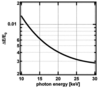Figure 3 Photon energy dependence of the lower limit for the spectral bandwidth, which can be provided behind prism-array monochromators according to equation (17)when made from SU8 photoresist.

3. Conclusion

It is shown that tunable refractive monochromators for X-rays can ultimately provide spectral bandwidths of below 1%. This is the lower limit and the bandwidth can always easily be adjusted to a larger bandwidth simply by opening the exit slit. Two concepts are discussed with acceptable averaged transmissions of the order of 44%: the use of off-axis prism-array lenses and the use of a composite prism in combination with a focusing mirror. The latter provides a smaller spectral bandwidth of the order of 0.3% for 15 keV photon energy and independent of the beam size, but it necessitates an additional focusing mirror which makes the beam transport more complicated. The former is already a monochromator. It is much more compact, however, with much less flexibility for the aperture and with a twofold larger bandwidth, i.e. with 0.6% at 15 keV photon energy. Compared with monochromators based on Si(111) crystals this is a 20- or 40-fold bandwidth increase, which translates here into a corresponding flux gain of about an order of magnitude. A more favorable material would be beryllium; however, presently the technology for fabricating the required microstructure is not available. By using it in a prism-array monochromator the lower limit for the bandwidth could be kept slightly below 0.2% in the photon energy range 10–30 keV. A composite prism in beryllium could then provide an interesting 0.1%. The reported performances are also feasible in combination with laboratory X-ray sources with sufficiently small source sizes.

Acknowledgements

I gratefully acknowledge very fruitful discussions with Dr Tao Liu from the Karlsruhe Institute of Technology.

ReferencesJOURNAL OFSYNCHROTRONRADIATION